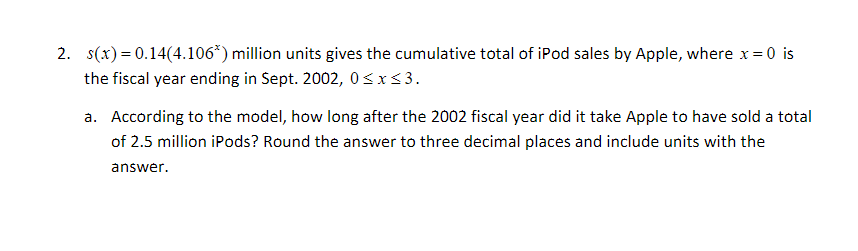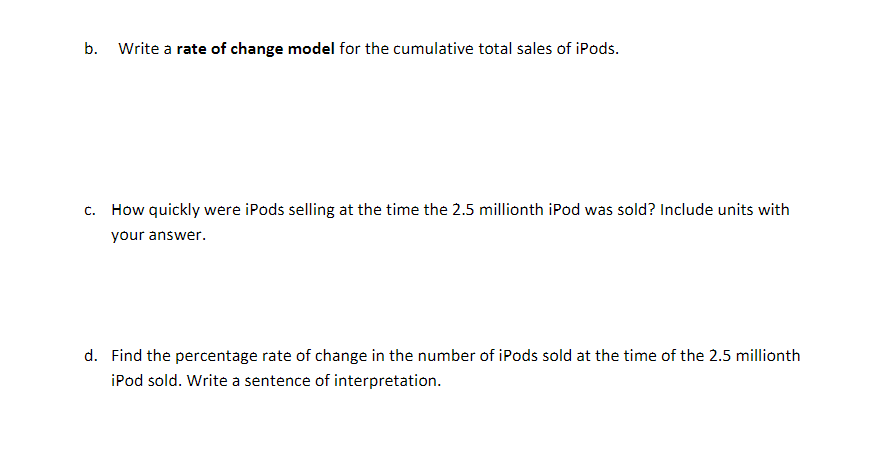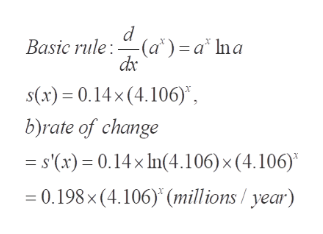# 2. s(x) 0.14(4.1063) million units gives the cumulative total of iPod sales by Apple, where x 0 is=the fiscal year ending in Sept. 2002, 0 xs3a. According to the model, how long after the 2002 fiscal year did it take Apple to have sold a totalof 2.5 million iPods? Round the answer to three decimal places and include units with theanswer

Question
15 views

i need help with part d)help_outlineImage Transcriptionclose2. s(x) 0.14(4.1063) million units gives the cumulative total of iPod sales by Apple, where x 0 is = the fiscal year ending in Sept. 2002, 0 xs3 a. According to the model, how long after the 2002 fiscal year did it take Apple to have sold a total of 2.5 million iPods? Round the answer to three decimal places and include units with the answer fullscreenfullscreen
check_circle

Step 1

As per norm, the first three questions are answered. To study the given model for s(x) and its derivative

Step 2

a) x = 2.041 years

Step 3

b) rate of change of a quantity is the derivative ...help_outlineImage TranscriptioncloseBasic rule:(a*) = a* Ina dac s(x) 0.14x(4.106)", b)rate of change s'(x) 0.14x In(4.106) x (4.106) = 0.198 x (4.106) (millions / year) fullscreen

### Want to see the full answer?

See Solution

#### Want to see this answer and more?

Solutions are written by subject experts who are available 24/7. Questions are typically answered within 1 hour.*

See Solution
*Response times may vary by subject and question.
Tagged in

### Derivative